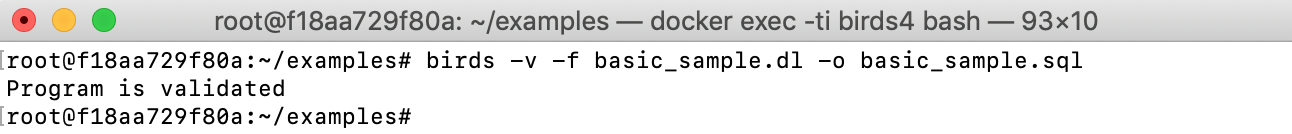View on GitHub BIRDS

# The basics

## The basic syntax

### Source and view schemas

Example: Consider two base tables `s1(X,Y)` and `s2(X,Y)` and a view `v(X,Y)`:

s1

X Y
1 2

s2

X Y
2 3

v

X Y
1 2
2 3

The source schema and the view schema are defined as follows:

``````source s1('X':int, 'Y':int).
source s2('X':int, 'Y':int).
view v('X':int, 'Y':int).
``````
• Using the keywords `source` and `view` to distinguish the source tables and the view.
• Each column is assigned a data type.
• Supported data types are: `integer`, `real` and `string`.

### View definition

`v` can be defined over `s1` and `s2` as follows:

``````v(X,Y) :- s1(X,Y).
v(X,Y) :- s2(X,Y).
``````

### Rules for update strategy:

• Delta predicate: a delta predicate is a normal predicate following a symbol `+` or `-`
• The predicate `+R` corresponds to the delta relation of tuples being inserted into source relation `R`
• The predicate `−R` corresponds to the delta relation of tuples being deleted from `R`
• Delta rule: a delta rule is a rule whose head is a delta predicate of a base table. For example, the following rule means any tuple `(X,Y)`, which is in `s1` but not in `v`, will be deleted from `s1`:

``````  -s1(X,Y) :- s1(X,Y), not v(X,Y).
``````

## An update strategy

The following is a full Datalog program for an update strategy on the view `v(X,Y)` (basic_sample.dl):

``````% schema:
source s1('X':int,'Y':int).
source s2('X':int,'Y':int).
view v('X':int,'Y':int).

% rules for update strategy:
-s1(X,Y) :- s1(X,Y), not v(X,Y).
-s2(X,Y) :- s2(X,Y), not v(X,Y).
+s1(X,Y) :- v(X,Y), not s1(X,Y), not s2(X,Y).
``````

The first two rules say that if a tuple `(X,Y)` is in `s1` or `s2` but not in `v`, it will be deleted from `s1` or `s2`, respectively. The last rule says that if a tuple `(X,Y)` is in `v` but in neither `s1` nor `s2`, it will be inserted to `s1`.

## Verifying and Compiling into SQL

We verify the correctness of the Datalog program and compile it into SQL by using command line `birds`:

``````birds -v -f basic_sample.dl -o basic_sample.sql
``````

Where the option `-v` enables verification process, `-f` is for the input Datalog program and `-o` is for the output SQL file.The generated SQL file can run directly in a PostgreSQL database to create the corresponding updatable view `v` in the database.

## Running in PostgreSQL

1. Load and run the generated SQL file in PostgreSQL:
`````` psql -U <db_user_name> -d <db_name> -f <path_to_sql_file>
``````

For example:

`````` psql -U postgres -d sample_db -f examples/basic_sample.sql
``````
2. Run an UPDATE/INSERT/DELETE statement to modify data on view, for example:
`````` INSERT INTO v VALUES (6,7);
``````
3. Request a bigger modification on view by combining multiple UPDATE/INSERT/DELETE statements in one transaction:
`````` BEGIN;
INSERT INTO v VALUES (6,7);
DELETE FROM v where x = 5;
UPDATE v SET x = 10 where x = 4;
END;
``````